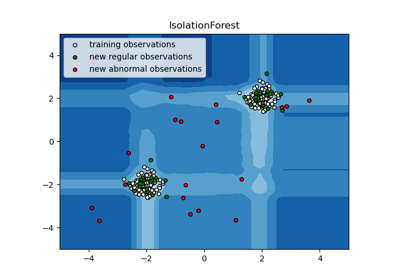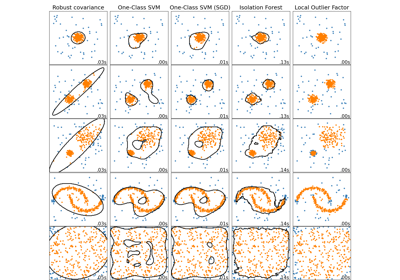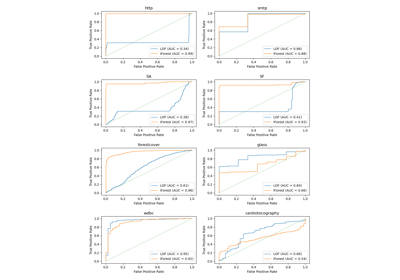# sklearn.ensemble.IsolationForest¶

class sklearn.ensemble.IsolationForest(*, n_estimators=100, max_samples='auto', contamination='auto', max_features=1.0, bootstrap=False, n_jobs=None, random_state=None, verbose=0, warm_start=False)[source]

Isolation Forest Algorithm.

Return the anomaly score of each sample using the IsolationForest algorithm

The IsolationForest ‘isolates’ observations by randomly selecting a feature and then randomly selecting a split value between the maximum and minimum values of the selected feature.

Since recursive partitioning can be represented by a tree structure, the number of splittings required to isolate a sample is equivalent to the path length from the root node to the terminating node.

This path length, averaged over a forest of such random trees, is a measure of normality and our decision function.

Random partitioning produces noticeably shorter paths for anomalies. Hence, when a forest of random trees collectively produce shorter path lengths for particular samples, they are highly likely to be anomalies.

Read more in the User Guide.

New in version 0.18.

Parameters:
n_estimatorsint, default=100

The number of base estimators in the ensemble.

max_samples“auto”, int or float, default=”auto”
The number of samples to draw from X to train each base estimator.
• If int, then draw max_samples samples.

• If float, then draw max_samples * X.shape samples.

• If “auto”, then max_samples=min(256, n_samples).

If max_samples is larger than the number of samples provided, all samples will be used for all trees (no sampling).

contamination‘auto’ or float, default=’auto’

The amount of contamination of the data set, i.e. the proportion of outliers in the data set. Used when fitting to define the threshold on the scores of the samples.

• If ‘auto’, the threshold is determined as in the original paper.

• If float, the contamination should be in the range (0, 0.5].

Changed in version 0.22: The default value of contamination changed from 0.1 to 'auto'.

max_featuresint or float, default=1.0

The number of features to draw from X to train each base estimator.

• If int, then draw max_features features.

• If float, then draw max(1, int(max_features * n_features_in_)) features.

bootstrapbool, default=False

If True, individual trees are fit on random subsets of the training data sampled with replacement. If False, sampling without replacement is performed.

n_jobsint, default=None

The number of jobs to run in parallel for both fit and predict. None means 1 unless in a joblib.parallel_backend context. -1 means using all processors. See Glossary for more details.

random_stateint, RandomState instance or None, default=None

Controls the pseudo-randomness of the selection of the feature and split values for each branching step and each tree in the forest.

Pass an int for reproducible results across multiple function calls. See Glossary.

verboseint, default=0

Controls the verbosity of the tree building process.

warm_startbool, default=False

When set to True, reuse the solution of the previous call to fit and add more estimators to the ensemble, otherwise, just fit a whole new forest. See the Glossary.

New in version 0.21.

Attributes:
base_estimator_ExtraTreeRegressor instance

The child estimator template used to create the collection of fitted sub-estimators.

estimators_list of ExtraTreeRegressor instances

The collection of fitted sub-estimators.

estimators_features_list of ndarray

The subset of drawn features for each base estimator.

estimators_samples_list of ndarray

The subset of drawn samples for each base estimator.

max_samples_int

The actual number of samples.

offset_float

Offset used to define the decision function from the raw scores. We have the relation: decision_function = score_samples - offset_. offset_ is defined as follows. When the contamination parameter is set to “auto”, the offset is equal to -0.5 as the scores of inliers are close to 0 and the scores of outliers are close to -1. When a contamination parameter different than “auto” is provided, the offset is defined in such a way we obtain the expected number of outliers (samples with decision function < 0) in training.

New in version 0.20.

n_features_int

DEPRECATED: Attribute n_features_ was deprecated in version 1.0 and will be removed in 1.2.

n_features_in_int

Number of features seen during fit.

New in version 0.24.

feature_names_in_ndarray of shape (n_features_in_,)

Names of features seen during fit. Defined only when X has feature names that are all strings.

New in version 1.0.

sklearn.covariance.EllipticEnvelope

An object for detecting outliers in a Gaussian distributed dataset.

sklearn.svm.OneClassSVM

Unsupervised Outlier Detection. Estimate the support of a high-dimensional distribution. The implementation is based on libsvm.

sklearn.neighbors.LocalOutlierFactor

Unsupervised Outlier Detection using Local Outlier Factor (LOF).

Notes

The implementation is based on an ensemble of ExtraTreeRegressor. The maximum depth of each tree is set to ceil(log_2(n)) where $$n$$ is the number of samples used to build the tree (see (Liu et al., 2008) for more details).

References



Liu, Fei Tony, Ting, Kai Ming and Zhou, Zhi-Hua. “Isolation forest.” Data Mining, 2008. ICDM’08. Eighth IEEE International Conference on.



Liu, Fei Tony, Ting, Kai Ming and Zhou, Zhi-Hua. “Isolation-based anomaly detection.” ACM Transactions on Knowledge Discovery from Data (TKDD) 6.1 (2012): 3.

Examples

>>> from sklearn.ensemble import IsolationForest
>>> X = [[-1.1], [0.3], [0.5], ]
>>> clf = IsolationForest(random_state=0).fit(X)
>>> clf.predict([[0.1], , ])
array([ 1,  1, -1])


Methods

 Average anomaly score of X of the base classifiers. fit(X[, y, sample_weight]) Fit estimator. fit_predict(X[, y]) Perform fit on X and returns labels for X. get_params([deep]) Get parameters for this estimator. Predict if a particular sample is an outlier or not. Opposite of the anomaly score defined in the original paper. set_params(**params) Set the parameters of this estimator.
decision_function(X)[source]

Average anomaly score of X of the base classifiers.

The anomaly score of an input sample is computed as the mean anomaly score of the trees in the forest.

The measure of normality of an observation given a tree is the depth of the leaf containing this observation, which is equivalent to the number of splittings required to isolate this point. In case of several observations n_left in the leaf, the average path length of a n_left samples isolation tree is added.

Parameters:
X{array-like, sparse matrix} of shape (n_samples, n_features)

The input samples. Internally, it will be converted to dtype=np.float32 and if a sparse matrix is provided to a sparse csr_matrix.

Returns:
scoresndarray of shape (n_samples,)

The anomaly score of the input samples. The lower, the more abnormal. Negative scores represent outliers, positive scores represent inliers.

property estimators_samples_

The subset of drawn samples for each base estimator.

Returns a dynamically generated list of indices identifying the samples used for fitting each member of the ensemble, i.e., the in-bag samples.

Note: the list is re-created at each call to the property in order to reduce the object memory footprint by not storing the sampling data. Thus fetching the property may be slower than expected.

fit(X, y=None, sample_weight=None)[source]

Fit estimator.

Parameters:
X{array-like, sparse matrix} of shape (n_samples, n_features)

The input samples. Use dtype=np.float32 for maximum efficiency. Sparse matrices are also supported, use sparse csc_matrix for maximum efficiency.

yIgnored

Not used, present for API consistency by convention.

sample_weightarray-like of shape (n_samples,), default=None

Sample weights. If None, then samples are equally weighted.

Returns:
selfobject

Fitted estimator.

fit_predict(X, y=None)[source]

Perform fit on X and returns labels for X.

Returns -1 for outliers and 1 for inliers.

Parameters:
X{array-like, sparse matrix} of shape (n_samples, n_features)

The input samples.

yIgnored

Not used, present for API consistency by convention.

Returns:
yndarray of shape (n_samples,)

1 for inliers, -1 for outliers.

get_params(deep=True)[source]

Get parameters for this estimator.

Parameters:
deepbool, default=True

If True, will return the parameters for this estimator and contained subobjects that are estimators.

Returns:
paramsdict

Parameter names mapped to their values.

property n_features_

DEPRECATED: Attribute n_features_ was deprecated in version 1.0 and will be removed in 1.2. Use n_features_in_ instead.

predict(X)[source]

Predict if a particular sample is an outlier or not.

Parameters:
X{array-like, sparse matrix} of shape (n_samples, n_features)

The input samples. Internally, it will be converted to dtype=np.float32 and if a sparse matrix is provided to a sparse csr_matrix.

Returns:
is_inlierndarray of shape (n_samples,)

For each observation, tells whether or not (+1 or -1) it should be considered as an inlier according to the fitted model.

score_samples(X)[source]

Opposite of the anomaly score defined in the original paper.

The anomaly score of an input sample is computed as the mean anomaly score of the trees in the forest.

The measure of normality of an observation given a tree is the depth of the leaf containing this observation, which is equivalent to the number of splittings required to isolate this point. In case of several observations n_left in the leaf, the average path length of a n_left samples isolation tree is added.

Parameters:
X{array-like, sparse matrix} of shape (n_samples, n_features)

The input samples.

Returns:
scoresndarray of shape (n_samples,)

The anomaly score of the input samples. The lower, the more abnormal.

set_params(**params)[source]

Set the parameters of this estimator.

The method works on simple estimators as well as on nested objects (such as Pipeline). The latter have parameters of the form <component>__<parameter> so that it’s possible to update each component of a nested object.

Parameters:
**paramsdict

Estimator parameters.

Returns:
selfestimator instance

Estimator instance.

## Examples using sklearn.ensemble.IsolationForest¶IsolationForest example

IsolationForest exampleComparing anomaly detection algorithms for outlier detection on toy datasets

Comparing anomaly detection algorithms for outlier detection on toy datasetsEvaluation of outlier detection estimators

Evaluation of outlier detection estimators